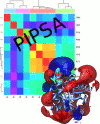> PIPSA (Protein Interaction Property Similarity Analysis)Version 3.2, November 2017

HITS gGmbH, Heidelberg, Germany
EMBL, Heidelberg, Germany

PIPSA may be used to compute and analyze the pairwise similarity of 3D interaction property fields for a set of proteins.

It is first described in:

N. Blomberg, R.R. Gabdoulline, M. Nilges and R. C. Wade.
Classification of protein sequences by homology modeling and quantitative analysis of electrostatic similarity.
Proteins: Str., Function and Genetics, 37:379-387 (1999).

The interaction properties are computed from the 3D coordinates of a set of superimposed proteins.
Similarity indices can be computed and analysed for three different types of property:
1.  The monopole and dipole moments of proteins, which are analytically computed descriptors for their electrostatic potentials;
2.  Electrostatic potentials obtained by numerically solving the finite-difference Poisson-Boltzmann equation (FDPBE) on a grid;
3.  Other types of interaction property, e.g. probe interaction energies evaluated with the GRID program, that can be mapped on to a grid.
PIPSA produces a matrix of pairwise similarity indices for each protein interaction property. This matrix is converted into a distance matrix which may  be used for clustering or visualization.

Theoretical Introduction

Short Overview

Privacy Imprint